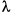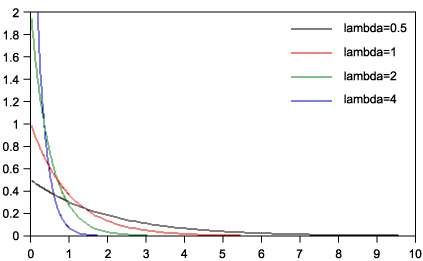Search

Useful Lists

Partners

Online Manuals

Apply probability models to Web data using PHP
By Paul Meagher - 2004-04-14 Page:  1 2 3 4 5 6 7 8 9 10 11

## And now, Exponential distribution

When probability modeling, you will not be using the probability distributions superclass directly. Instead, you will be interacting with specific instantiations of the probability distributions superclass, one for each common probability distribution for which analytic work has been done to figure out how to implement the PDF, CDF, InverseCDF, and RNG methods.

R, for example, includes such methods for all of these univariate distributions:

• Beta
• Binomial
• Cauchy
• Chi Square
• Exponential
• F
• Gamma
• Geometric
• Hypergeometric
• Log-Normal
• Logistic
• Negative Binomial
• Poisson
• Student's t
• Uniform
• Weibull
• Wilcoxon

As you can see, when it comes to fitting the probability distribution for your random variable to a specific theoretical probability distribution, you can choose from many such distributions. For you to become proficient in basic probability modeling, you need to become familiar with:

1. The visual representation of such distributions
2. The shape and location adjustment parameters that most probability distributions accept
3. Which adjustment parameters are used for model fitting

Statistics textbooks and Web sites can be consulted for these details. See Resources.

I want to focus on one particular probability distribution called the exponential distribution. What you learn from this example can be used to understand how to use other probability distributions to construct univariate probability models.

The exponential distribution in particular possesses these four advantages:

• The implementation of the distribution function methods can be easily found in textbooks or online so that you can see where they came from. Many math books focus on using positive and negative exponential functions to model change in some variable (for example, balance) as a function of time (for example, term interest rate).
• The exponential distribution can be used to construct basic probability models (such as assigning probabilities to various statements about a random variable) for the behavior of a large number of phenomenon (for example, random variables of interest). It is commonly used to model the distribution of waiting times -- either the time elapsed until some event occurs or the interval between each occurrence of an event. I will illustrate such an application in the next section.
• Textbooks on applied probability modeling often focus on the Exponential and Poisson distributions in particular because together they have demonstrated general utility and can be used to construct more elaborate probability models. In other words, not all probability distributions are equivalent in terms of their usefulness in modeling a variety of real-world phenomenon. The Exponential and Poisson distributions in particular are extremely useful for applied, real-world probability modeling. The Uniform, Normal and Binomial probability distributions are three other distributions you should make an effort to study in detail.
• When the observed distribution of your random variable is exponentially distributed, understanding the likelihood of different outcomes is much harder to do by intuition, so it helps in these cases especially to have a formal probability model for the random variable.

Start looking at the Exponential distribution by seeing some examples. The shape and location aspects of the Exponential distribution are determined by a single parameter called lambda (, also known as the rate or decay parameter). Figure 6 plots the probability distributions for four different values of lambda.

Figure 6. Four lambda probability distributionsListing 4 is the code that implements the probability distribution methods for an Exponential distribution. Notice that the PDF method returns probability values for given values of X; this information was used to construct the lines in Figure 6. Also notice that the core distribution methods (`PDF()`, `CDF()`, `InverseCDF()`, and `RNG()`) return a single answer or a vector of answers depending on how the method is called. This feature will be demonstrated later in a script that tests the methods of this class.

Listing 4. Implementing probability distribution methods for an Exponential distribution
 `````` rate = \$decay; } function Mean() { return \$this->rate; } function Variance() { return \$this->rate * \$this->rate; } function PDF(\$x) { if (is_array(\$x)) { \$pdf_vals = array(); \$num_vals = count(\$x); for(\$i=0; \$i < \$num_vals; \$i++) { if (\$x[\$i] < 0.0) { return PEAR::raiseError("Input values must be greater than 0."); } \$pdf_vals[\$i] = \$this->rate * exp(-\$this->rate * \$x[\$i]); } return \$pdf_vals; } else { if (\$x < 0.0) { return PEAR::raiseError("Input value must be greater than 0."); } \$pdf_val = \$this->rate * exp(-\$this->rate * \$x); return \$pdf_val; } } function CDF(\$x) { if (is_array(\$x)) { \$cdf_vals = array(); \$num_vals = count(\$x); for(\$i=0; \$i < \$num_vals; \$i++) { if (\$x[\$i] < 0.0) { return PEAR::raiseError("Input values must be greater than 0."); } \$cdf_vals[\$i] = 1.0 - exp(-\$this->rate * \$x[\$i]); } return \$cdf_vals; } else { if (\$x < 0.0) { return PEAR::raiseError("Input value must be greater than 0."); } \$cdf_val = 1.0 - exp(-\$this->rate * \$x); return \$cdf_val; } } function InverseCDF(\$prob) { if (is_array(\$prob)) { \$inv_vals = array(); \$num_vals = count(\$prob); for(\$i=0; \$i < \$num_vals; \$i++) { if (\$this->checkRange(\$prob[\$i])) { return PEAR::raiseError("Probability values must be between 0.0 and 1.0"); } \$inv_vals[\$i] = -log(1.0 - \$prob[\$i]) / \$this->rate; } return \$inv_vals; } else { if (\$this->checkRange(\$prob)) { return PEAR::raiseError("Probability value must be between 0.0 and 1.0"); } \$inv_val = -log(1.0 - \$prob) / \$this->rate; return \$inv_val; } } function RNG(\$num_vals=1) { if (\$num_vals < 1) { return PEAR::raiseError("Number of random values must be greater than 0"); } if (\$num_vals == 1) { \$rand_val = mt_rand() / mt_getrandmax(); return -log(\$rand_val) / \$this->rate; } else { \$rand_vals = array(); for(\$i=0; \$i < \$num_vals; \$i++) { \$rand_val = mt_rand() / mt_getrandmax(); \$rand_vals[\$i] = -log(\$rand_val) / \$this->rate; } return \$rand_vals; } } } ?> ``````

To gain insight into how this class is used and what it does, I have created a script in the tests directory of the accompanying `PHPMath_ProbabilityDistribution` package that demonstrates usage and method-return values. The script is called exponential.php and looks like this:

Listing 5. The exponential.php script demonstrates usage and method-return values
 `````` Mean(); \$Output = \$exp->Variance(); \$Output = \$exp->PDF(2); \$Output = \$exp->CDF(3); \$Output = \$exp->InverseCDF(0.95); \$Output = \$exp->RNG(1); make_table("Exponential Distribution (lambda=1)", "Methods", "Output", \$Methods, \$Output); // Test PDF function by feeding an array of \$x_vals and // getting a corresponding array of \$p_vals. \$X_Vals = array(0.0, 0.5, 1.0, 1.5, 2.0, 2.5, 3.0, 3.5); \$P_Vals = \$exp->PDF(\$X_Vals); make_table("PDF(X_VALS)", "X Vals Input", "P Vals Output", \$X_Vals, \$P_Vals); // Test CDF function by feeding an array of \$x_vals and getting // a corresponding array of \$p_vals where each p_val corresponds // to p(x < \$x_vals[\$i]) \$X_Vals = array(0.0, 0.5, 1.0, 1.5, 2.0, 2.5, 3.0, 3.5); \$P_Vals = \$exp->CDF(\$X_Vals); make_table("Vector Test of CDF(X_VALS)", "X Vals Input", "P Vals Output", \$X_Vals, \$P_Vals); // Test InverseCDF function by feeding in P_Vals from previous // test. Result should be mirror of CDF output. \$X_Vals = \$exp->InverseCDF(\$P_Vals); make_table("InverseCDF(P_VALS)", "P Vals Input", "X Vals Output", \$P_Vals, \$X_Vals); // Test RNG function by passing the number of values you want generated. // Result is an array of random numbers from an exponential distribution \$Counter = range(0, 7); \$Rnd_Vals = \$exp->RNG(8); make_table("RNG(N_VALS)", "Counter", "Rnd Vals", \$Counter, \$Rnd_Vals); ?> ``````

The exponential.php test script generates five HTML tables.

Table 2 demonstrates the single values that all the methods in the `Exponential.php` class can return. You can verify that these methods are producing accurate results by comparing their output to what a more mature statistical computing environment like R would generate (for an example, revisit the `dexp()`, `pexp()`, `qexp()`, and `rexp()` functions).

Table 2. Single values all methods in Exponential.php returns

 Exponential Distribution (lambda=1) Methods Output Mean() 1 Variance() 1 PDF(2) 0.13533528323661 CDF(2) 0.95021293163214 InverseCDF(0.95) 2.995732273554 RNG(1) 2.4945071670198

You can also use these tables to assist you in understanding the types of values the functions return when passed different arguments. Tables 3, 4, and 5 are particularly useful for this. They illustrate the vector orientation of these methods and consequently allow you to see the behavior of the three major distribution functions (PDF, CDF, and InverseCDF) over a range of input values (when lambda is set to 1).

 PDF(X_VALS) X Vals Input P Vals Output 0 1 0.5 0.60653065971263 1 0.36787944117144 1.5 0.22313016014843 2 0.13533528323661 2.5 0.082084998623899 3 0.049787068367864 3.5 0.030197383422319

 CDF(X_VALS) X Vals Input P Vals Output 0 0 0.5 0.39346934028737 1 0.63212055882856 1.5 0.77686983985157 2 0.86466471676339 2.5 0.9179150013761 3 0.95021293163214 3.5 0.96980261657768

 InverseCDF(P_VALS) P Vals Input X Vals Output 0 0 0.39346934028737 0.5 0.63212055882856 1 0.77686983985157 1.5 0.86466471676339 2 0.9179150013761 2.5 0.95021293163214 3 0.96980261657768 3.5

Table 6 displays output from the random-number generator. The `RNG()` method generates exponentially distributed random numbers using the value of lambda to adjust the shape and location aspects of the exponential distribution you are sampling from.

Table 6. Output from the random-number generator

 RNG(N_VALS) Counter Rnd Vals 0 1.658002921542 1 2.0097168931621 2 0.41562004896617 3 1.9121062991322 4 0.21394501966731 5 0.8054845401079 6 0.50914083865687 7 1.2056725040028

View Apply probability models to Web data using PHP Discussion

Page:  1 2 3 4 5 6 7 8 9 10 11 Next Page: Building a probability model